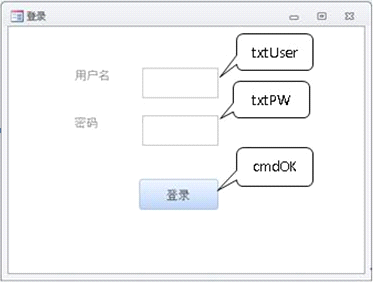#### 登录窗体如图所示。单击"登录"按钮，当用户名及密码正确时则会弹出窗口显示"OK"信息。 下列过程不能完成此功能的是• A.Private Sub cmdOK_Click() If txtUser.Value = "zhangs" And txtPW.Value = "123" Then MsgBox "OK" End if End Sub
• B.Private Sub cmdOK_Click() If txtUser.Value = "zhangs" Then If txtPW.Value = "123" Then MsgBox "OK" End if End if End Sub
• C.Private Sub cmdOK_Click() If txtUser.Value = "zhangs" Then If txtPW.Value = "123" Then MsgBox "OK" End if End Sub
• D.Private Sub cmdOK_Click() If txtUser.Value = "zhangs" and txtPW.Value = "123" Then MsgBox "OK" End If End Sub

【解析】通常，一行之中有一条语句，而没有语句终结符，但是也可以将两个或者多个语句放在同一行，并用冒号"："隔开，这称之为复合。A选项一行字有两条语句，但未用冒号隔开，所以A选项不能实现。因此，本题应选择A选项。Emoji表情贴图
DIY有趣的emoji表情